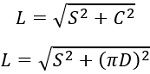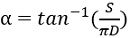# Helical Antenna

Definition: Helical antenna is a type of antenna that is designed using conducting wire and is constructed in the shape of a helix. These antennas are considered as one of the simplest antennas and are widely used in very high and ultra-high frequency operations.

So, finds applications in extraterrestrial communication like satellite relays etc.

The operating frequency of helical antennas lies between 300 MHz to 3 GHz.

Generally, helical antennas radiate circularly polarized waves and support two modes of operations, which are:

• Normal mode
• Axial mode

## Content: Helical Antenna

### Construction

A helical antenna is constructed using a conducting wire. Generally, a thick copper wire is wound in the form of a helix and is used in conjunction with a metallic plate. This metallic plate is the ground plate.

The excitation to the helical antenna is provided at an end using a coaxial transmission line. Here the central conductor is attached to the helix and outer conductor forms a connection with the ground plane. The figure below represents the structure of the helical antenna:Generally, a sheet and concentric conductor is used to form the ground plane.

We have already discussed in the beginning that there are two modes of operation of the helical antenna. And the deciding factor for the mode of operation is the diameter of the helix and spacing between two consecutive turns.

Suppose for a helical antenna, the length of a single turn is denoted as L while S denotes the spacing between the consecutive turns. Then the total length of the antenna will be NS.

The circumference of the helix is denoted as C and is given as πD.

: D represents the diameter of the helical spring.

Consider unrolling one turn of the helix, then the various parameters associated with it can be defined by the triangle given below:Here α is the pitch angle

Therefore, we will have:The pitch angle of the helix is the angle existing between the tangential line to the helical wire and the plane in direction, normal to its axis.

ThusHenceThus, we can say, the radiation characteristics of the antenna are changed by varying these parameters according to the wavelength.

## Working of Helical Antenna

We know that when a conductive wire is excited by using feed likes like coaxial cable or two-wire transmission line then current flows through the conducting wire. This causes the generation of field lines and radiations are emitted.

It is noteworthy that a helical antenna may radiate in various mode but the two most prominent modes are discussed here.

This mode of operation is also known as perpendicular mode of operation, this is so because, in this mode, maximal radiation is emitted in the direction of the plane perpendicular to the axis of the antenna. And the waves are circularly polarized in nature. This means that the field radiated is maximum along the plane perpendicular to axis and minimum along the axis.

This mode of operation is achieved when the dimensions of the antenna are comparatively smaller than the wavelength. This means

NL < < λ

However, this small dimensional antenna offers low radiation efficiency and very narrow bandwidth.

The antenna in this configuration is known as broadside helical antenna and acts as an electric short dipole antenna.

It is to be noted that a helix resembles like a combination of small loop and short dipole. Thus its radiation pattern resembles the radiation pattern of the combination of a short dipole and small loop placed along the axis of the helix.

The reason behind this is that at α = 0°, the helix corresponds as loop and at α = 90°, it acts as a linear dipole. Therefore, patch angle at 0° and 90° serves as the limiting conditions of the helical antenna.

So, we can say, if the spacing tends to zero but the diameter is constant then helix resembles like a loop. But if the spacing is fixed while diameter tends to zero, then helix transforms into a linear conductor.

This is represented below:Due to the compact structure of this antenna, it offers suitability of use on mobile and portable devices operating on VHF and UHF bands.

Axial mode is also referred as the end-fire mode of operation as here the maximum radiation emission occurs along the axis of the helix. The radiation pattern of the end-fire helical antenna is circular or nearly circular.

The crucial differentiating factor between normal mode and axial mode is that in normal mode, maximum radiation is along the perpendicular direction of the axis. While in axial mode, maximum radiation is along the axis itself.

Now the question arises what is the condition of having an axial mode of operation?

So basically, this mode of the helical antenna is the result of such dimensions where the circumference and the spacing between the loops are somewhat equivalent to one wavelength.

This means,

NL ≈ λ

Unlike normal mode, this mode of the helical antenna produces a broad and highly directional beam in the axial direction. Thus, this mode of the helical antenna is used for practical applications.

For practical purpose, the helix is made with circumference nearly equal to one wavelength and spacing nearly λ/4.

For axial mode, the pitch angle shows variation between 12° to 18°, however, at 14° this mode shows best results.

The complete length of the helix decides its gain and bandwidth.

The value of terminal impedance is nearly 100 ohms and varies according to frequency.

The figure below represents the radiation pattern of the helical antenna operating in axial mode:The various advantages of the helical antenna are:

1. These offer simple construction.
2. The helical antenna exhibits highly directional behaviour.
3. It offers wide operating bandwidth.
4. The operational frequency range is very high i.e., operates in VHF and UHF.

The drawbacks associated with helical antennas are as follows:

1. The end-fire helical antenna exhibit large size thus is bulky.
2. The efficiency of the antenna is dependent on the number of turns, as with the increase in the number of turns, efficiency decreases.

### Applications of Helical Antenna

Helical antennas majorly find applications in space communication. Their simplicity, high directivity, wide bandwidth and circular polarization serve as the parameters for operating in space communication.

Generally, the telemetry data in space communication is propagated through helical antennas.

Also, by placing either single or array of the helical antenna in the surface, ionospheric wave propagation can be achieved. This allows the transmission and reception of VHF signals. Thus, satellite and space probe propagation use helical antennas.

Helical antennas configured in axial mode can receive signals of arbitrary polarization easily. As end-fire helical antennas offer circularly polarized waves over a wider bandwidth. Hence is widely used than broadside helical antennas.